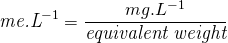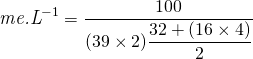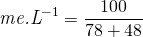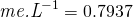The amount of nutrients in a solution is expressed as a concentration. For instance; if 1 g of potassium sulphate is added to 1,000 L of water, one could express the amount of potassium sulphate in relation to the amount of water as 1 g.1000 L-1 potassium sulphate solution (it can also be expressed as 1 g/1000 L potassium sulphate solution). Converting the grams to milligram would result in 1000 mg.1000 L-1 potassium sulphate solution. Any value provided in mg per L is equal to parts per million. Thus 1 mg.L-1 = 1 ppm and 100 mg.L-1 = 100 ppm.

Milli equivalent is the preferred method of expressing the concentration of an element in water. In order to calculate the equivalent values, the molecular weight of each element needs to be altered. The equivalent weight of a formula is the atomic weight or molecular weight divided by its valence. The valence is the sign, negative or positive, of the element or formula as it is found in solution.

If we take the potassium sulphate (K2SO4) molecule as an example: Converting from 100 mg.L-1 potassium sulphate (K2SO4) to me.L-1 we would use the following equation.Thus 100 mg.L-1 potassium sulphate is equal to 0.7937 me.L-1. When calculating ratio’s of elements in a nutrient solution, the milli equivalent value is used and not parts per million (ppm) or milligrams (mg).